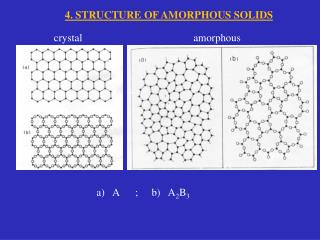DownloadDownload Presentationcrystal

crystal

Télécharger la présentationcrystal

- - - - - - - - - - - - - - - - - - - - - - - - - - - E N D - - - - - - - - - - - - - - - - - - - - - - - - - - -
Presentation Transcript

1. 4. STRUCTURE OF AMORPHOUS SOLIDS crystal amorphous a) A ; b) A2B3

2. coordination number z gives some hints: • A low coordination number (z = 2, 3, 4) provides evidence for a dominant role of covalent bonding (SiO2, B2O3…) • More “closed-packed” structures are symptomatic of non-directional forces (ionic, van der Waals, metallic bonding…): z(NaCl)=6, z(Ca)=8, z(F)=4 … • fcc or hcp structures are typical of metallic crystals AB forming a close-packed lattice with z=12, the extreme of maximum occupation.

3. RDF Radial Distribution Function J(r) = 4 r 2 (r)

4. RDF J (r) = 4 r 2 (r)

5. 3 main kinds of atomic-scale structure (models) of amorphous solids: • Continuous Random Network  covalent glasses • Random Close Packing  simple metallic glasses • Random Coil Model  polymeric organic glasses

6. Amorphous Morphology: Continuous Random Network. crystals amorphous a) A ; b) A2B3 Continuous Random Network (Zachariasen, 1932)

7. Amorphous Morphology. Amorphous Morphology: Continuous Random Network. - coordination number COMMON: - (approx.) constant bond lengths - ideal structures (no dangling bonds…) DIFFERENT: - significant spread in bond angles - long-range order is absent

8. Review of crystalline close packing.

9. Review of crystalline close packing. Calculate the packing factor for the FCC cell: In a FCC cell, there are four lattice points per cell; if there is one atom per lattice point, there are also four atoms per cell. The volume of one atom is 4πr3/3 and the volume of the unit cell is .

10. Amorphous Morphology: Random Close Packing There is a limited number of local structures. The volume occupancy is 64%

11. Amorphous Morphology: Random Coil Model RCM is the most satisfactory model for polymers, based upon ideas developed by Flory (1949, …, 1975). Each individual chain is regarded as adopting a RC configuration (describable as a 3-D random walk). The glass consists of interpenetrating random coils, which are substantially intermeshed – like spaghetti !!!

12. Basic geometry for diffraction experiments: DIFFRACTION EXPERIMENTS = h c / E I (k) = h / (2·m·E)1/2 k = (4 / ) sen 

13. Neutron scattering It allows to take data to higher values of k (using smaller wavelengths) and hence reduce “termination errors” in the Fourier transform. Neutrons emerge from a nuclear reactor pile with   0.11 Å Scattering events: Energy transfer: Momentum transfer: Scattering function: